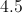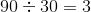# SSAT Middle Level Math : How to find the part from the whole

## Example Questions

← Previous 1 3 4 5 6 7 8 9 10 11 12

### Example Question #1 : How To Find The Part From The Whole

It took Julia 15 minutes to walk 1 mile.  If she walks for 2 more miles at the same speed, what part of an hour will her entire 3-mile walk take?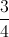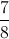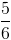Explanation:

It will take her 30 more minutes to finish her walk for a total of 45 minutes.  45 minutes of 60 minutes in an hour is the same as.

### Example Question #2 : How To Find The Part From The Whole

The sum of the factors of 12 is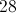Explanation:

The factors of 12 are 1, 12, 3, 4, 2, and 6.  The sum of them is when you add all of them together.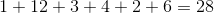.

### Example Question #3 : How To Find The Part From The Whole

There arechildren in a class. Ifof the children are male, how many of them are female?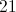Explanation:

Ifare male, thenof the children in the class are female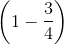.

If this is true, thentimes the total number of students in the class will give you the number of females.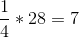### Example Question #1 : How To Find The Part From The Whole

The ratio of women to men in a meeting with 56 people is 5 to 3.  How many more women then men are there?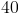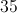Explanation:

The best way to think about this problem is to divide the total number of people into parts. Since there are 5 parts women to 3 parts men, it's best to divide the total into 8 parts.  Dividing 56 into 8 parts gives you 7.  7 is your multiplier.  Now take your multiplier (7) and multiply it by your ratios.

This give you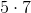and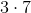orand.  These are the number of women and men, respectively.

The difference between 35 and 21 is 14.

### Example Question #1 : How To Find The Part From The Whole

Robert's dog is 4 years older than Karen's cat. In 3 years, the sum of the ages of Robert's dog and Karen's cat will be 13. How old is Robert's dog right now?years oldyears oldyears oldyears oldyears oldyears old

Explanation:

We can solve this problem by backsolving, meaning plugging in the answer choices.

If we plug in 7 for the dog's age, that means that in 3 years, the dog will be 10.

That also means that the cat is 3 since the dog is 4 years older.

The sum of 10 and 3 is 13, so the answer choice of 7 is the correct answer.

### Example Question #6 : How To Find The Part From The Whole

There arepuppies in obedience training. Ifof the puppies are male, how many of them are female?Explanation:

Ifare male, thenof the puppies in obedience training are female. Because: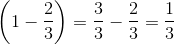.

Now we can write the following proportion and solve.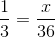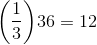### Example Question #7 : How To Find The Part From The Whole

The ratio of doctors to nurses in the hospital with a staff of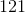isto. How many more nurses than doctors are there?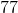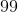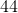Explanation:

The best way to think about this problem is to divide the total number of people into parts. Since there areparts doctors toparts nurses, it's best to divide the total intoparts. Dividingintoparts gives you. Therefore,is your multiplier. Now take your multiplier and multiply it by your ratios.

This gives you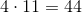and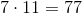. These are the number of doctors and nurses, respectively.

To find how many more nurses than doctors there are we take the difference: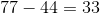Therefore, there aremore nurses than doctors.

### Example Question #42 : Numbers And Operations

Alice received $2.40 for her weekly allowance. If her favorite snacks cost 60¢ each, how many snacks can Alice buy this week? Possible Answers:Correct answer:Explanation: To solve: Divide the total amount of Alice's allowance ($2.40) by the price for each snack (\$ .60)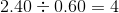Alice would be able to buy 4 snacks.

### Example Question #1 : How To Find The Part From The Whole

Steve ate 20% of his pie on Monday.  On Tuesday Steve ate 50% of the left-over pie.  What percent of the pie is left?

10%

60%

30%

40%

20%

40%

Explanation:

On Tuesday, Steve ate 50% (half) of the 80%, which is 40%.  Steve has eaten 20% on Monday and 40% on Tuesday, so there is 40% left over.

### Example Question #43 : Numbers And Operations

As a swim team member, Nicolas needs to train 90 hours a month. How many hours should Nicolas train each day of the 30-day month, in order to reach his goal?